# Very Short Answer Type Questions- Surface Areas and Volumes Class 10 Notes | EduRev

## Class 10 : Very Short Answer Type Questions- Surface Areas and Volumes Class 10 Notes | EduRev

The document Very Short Answer Type Questions- Surface Areas and Volumes Class 10 Notes | EduRev is a part of the Class 10 Course Mathematics (Maths) Class 10.
All you need of Class 10 at this link: Class 10

Q1. A cylinder, a cone and a hemisphere have the same values for r and h. Find the ratio of their volumes.

Sol. Let radius be ‘r’ and height ‘h’.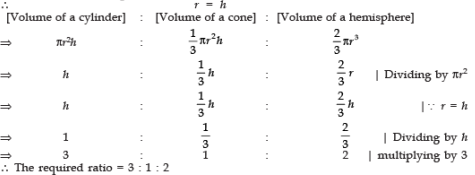Q2. If two identical solid cubes of side ‘x’ are joined end to end, then the total surface area of the resulting cuboid is 12x2. Is it true?

Sol. ∵ The total surface area of a cube of side x is 6x2
When they are joined end to end, the lenght bcomes 2x
∴Total surface area
= 2[lh + bh + hl]
= 2 [(2x · x) + (x · x) + (2x · x)]
= 2 [2x2 + x2 + 2x2]
= 2 [5x2] = 10x2 ≠ 12x2
∴ False

Q3. A spherical ball is melted to make eight new identical balls. Then the radius of each new ball is 1/8 th of the radius of the original ball. Is it true?

Sol. ∵ Radius ‘R’ of original ball

⇒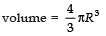And Radius ‘r’ of the new ball

⇒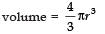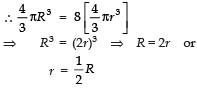⇒ Radius of the new ball
= Half the radius of original ball.
∴ False

Q4. If a solid cone of base radius ‘r’ and height ‘h’ is placed over a solid cylinder having same base radius ‘r’ and height - ‘h’ as that of the cone, then the curved surface area of the shapeis Is it true?

Sol. ∵ Curved surface area of a cone =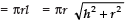And curved surface area of the cylinder = 2πrh
∴ The curved surface area of the combination  = πr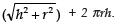∴ True.

Q5. A cylinder and a cone are of the same base radius and same height. Find the ratio of the volumes of the cylinder of that of the cone.

Sol. Let the base radius = r and height = h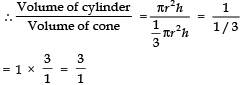⇒ The required ratio = 3 : 1

Offer running on EduRev: Apply code STAYHOME200 to get INR 200 off on our premium plan EduRev Infinity!

## Mathematics (Maths) Class 10

178 videos|282 docs|103 tests

,

,

,

,

,

,

,

,

,

,

,

,

,

,

,

,

,

,

,

,

,

;